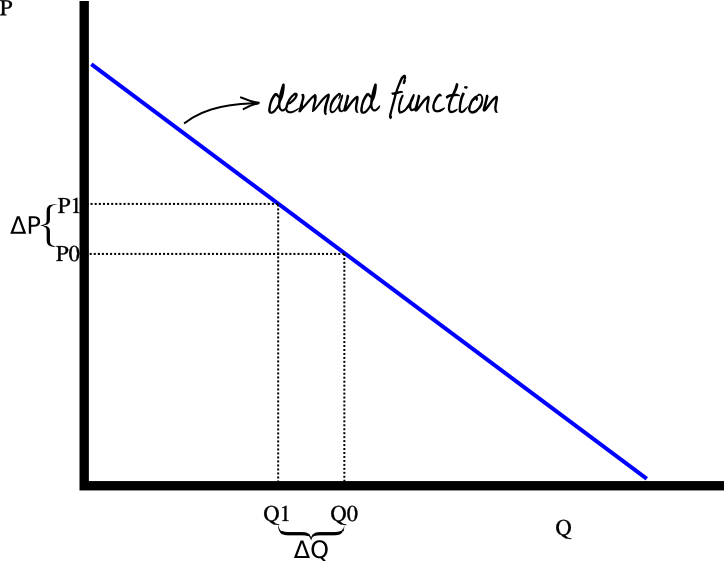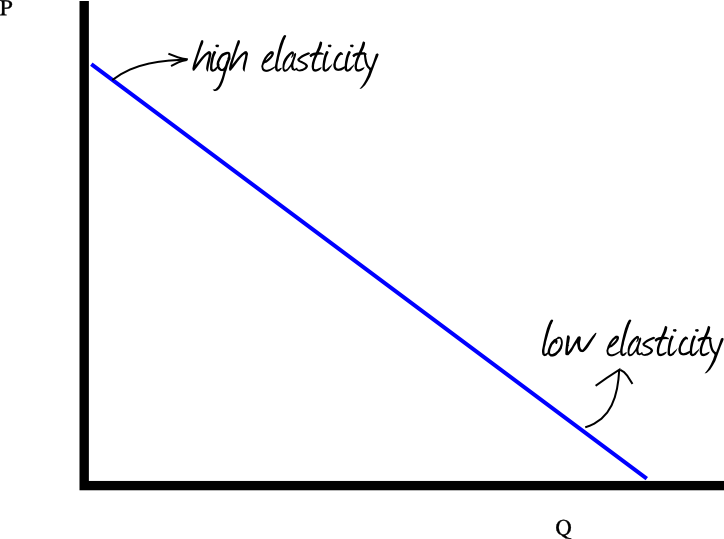### Site Tools

en:price-elasticity-of-demand

# Price Elasticity of the Demand

It is the relative change of the quantity demanded in relation to the relative change of the price.

ΔQd/Qd / ΔP/P

Graphically:Usually, when the price increases, the quantity demanded decreases. Thus, the price elasticity of the demand is negative.

The value of the elasticity changes along the same demand function. For example, if the quantity is next to 0, the elasticity will be high. Over the same demand function, if the price is next to 0, the elasticity will be high.:FINISH ME:

## Discussion

Enter your comment. Wiki syntax is allowed:
W Y E﻿ V R